# Random and pseudo-random numbers

Numbers(in particular, binary digits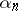) whose sequential appearance satisfies some kind of statistical regularity (see Probability theory). One distinguishes random numbers, being generated by a stochastic apparatus, and pseudo-random numbers, being constructed using arithmetical algorithms. It is usually assumed (for better or worse reason) that the sequence obtained (or constructed) has frequency properties that are "typical" for a sequence of independent realizations of some random variablewith distribution function; then one speaks of (independent) random numbers distributed according to the law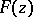. The most commonly used examples are as follows: random numbers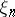uniformly distributed on the interval,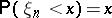, equi-probable random binary digits,, and random normal numbers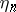distributed according to the normal law with meanand variance 1 (cf. Uniform distribution; Normal distribution). Random numbers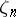with an arbitrary distribution functioncan be constructed from a sequence of uniformly-distributed random numbersby putting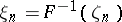, that is, they can be found from the equation,. There are also other methods of construction: for example, it is analytically simpler to obtain normally-distributed random numbers from uniformly-distributed random numbers by using the pairs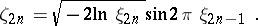Random and pseudo-random numbers are used in practice in the theory of games (cf. Games, theory of), in mathematical statistics, in Monte-Carlo methods (cf. Monte-Carlo method), and in cryptography, for the concrete realization of non-determined algorithms and behaviour predicted only "on the average" . For example, if the next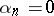, then a player chooses the first strategy, but if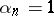, then he/she takes the second.
It is only possible to attach a strict mathematical sense to the concept of random numbers in the framework of the algorithmic probability theory of A.N. Kolmogorov  and P. Martin-Löf . Letbe the countably-dimensional unit hypercube, letbe the Lebesgue measure onand let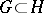be a largest constructively-described measurable set of measure zero (it exists). Then any sequencecan be regarded as typical, and so taken as a sequence of uniformly-distributed random numbers. Similarly one can introduce the concept of constructive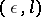-typicality of an-sequence of binary symbols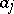,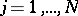, with respect to the system of all events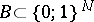: to be of measure not more thanand of length of description not more than. It is evident from the definition that a typical sequence of uniformly-distributed random numbers cannot itself be constructive, and even the construction of an-typical sequence of random symbols requires an extraordinary large search. Therefore, in practice one uses simpler algorithms, allowing checking of their statistical "quality" with a few tests. Thus, in constructing uniformly-distributed random numbers one must necessarily test the uniform distribution of the sequence (see ). In simple problems, the fulfillment of certain tests can actually guarantee the usefulness of a sequence. It is sometimes more effective to use correlated random numbers, constructed from a sequence of uniformly-distributed random numbers.